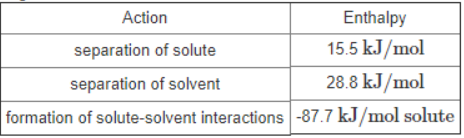# Problem: A hypothetical solution forms between a solid and a liquid. The values of the thermodynamic quantities involved in the process are shown in the following table. Calculate the enthalpy of solution in kilojoules per mole of solute. Enter your answer numerically in kilojoules per mole of solute.

###### FREE Expert Solution

Recall that ΔH˚rxn can be calculated from the enthalpy of formation (ΔH˚f) of the reactants and products involved:

$\overline{){\mathbf{∆}}{\mathbf{H}}{{\mathbf{°}}}_{{\mathbf{rxn}}}{\mathbf{=}}{\mathbf{∆}}{\mathbf{H}}{{\mathbf{°}}}_{\mathbf{f}\mathbf{,}\mathbf{products}}{\mathbf{-}}{\mathbf{∆}}{\mathbf{H}}{{\mathbf{°}}}_{\mathbf{f}\mathbf{,}\mathbf{reactants}}}$

92% (448 ratings)###### Problem Details

A hypothetical solution forms between a solid and a liquid. The values of the thermodynamic quantities involved in the process are shown in the following table.Calculate the enthalpy of solution in kilojoules per mole of solute. Enter your answer numerically in kilojoules per mole of solute.